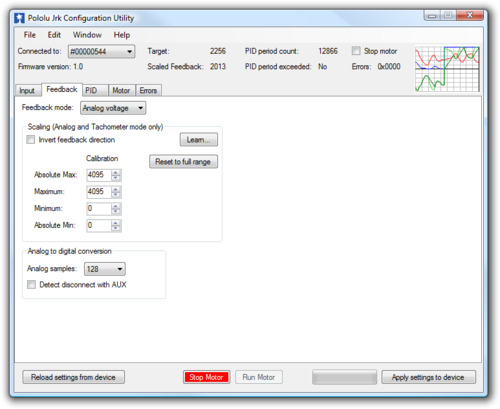# 3.c. Feedback Options

The Feedback tab of the Jrk Configuration UtilityThe Feedback tab of the jrk configuration utility controls the measurements of the output of the control system. If this section is properly configured, the value of scaled feedback will be 0 when the output is at the minimum position and 4095 when the output is at its maximum. There are three available feedback modes:

• None indicates that feedback and the PID calculation are disabled. In this mode, the duty cycle target is equal to target − 2048 instead of being the result of a PID calculation. This means that a target of 2648 will correspond to driving the motor full speed forward, 2048 is brake, and so on. However, the jrk still performs all of its calculations once per “PID period”.
• Analog voltage is used when an analog voltage source, such as a potentiometer, connected to the FB pin indicates the position of the output. A signal level of 0 V corresponds to a feedback value of 0, and a signal level of 5 V corresponds to a feedback value of 4092.
• Frequency (digital) is used with speed-measuring devices that generate pulses at a rate proportional to the speed of the output shaft, such as a tachometer. A simple example is an optical breakbeam sensor measuring the rotation of a slotted disk. The number of pulses detected on the FB pin during each PID period is used as a measurement of speed. When driving the motor forward (i.e. target > 2048), the feedback value is 2048+n, where n is the number of pulses, and when driving the motor in reverse, the feedback value is 2048-n. Since the feedback value must be between 0 and 4095, the jrk can measure at most 2047 pulses per PID period. This allows for a maximum frequency of approximately 2 MHz with a PID period of 1 ms.

#### Feedback scaling

The scaling options in this tab determine how the raw feedback values map to scaled feedback values, which are intended to be a representation of the output of the system. The parameters Maximum and Minimum should be set to the maximum and minimum possible values of the output; these will be converted to scaled feedback values of 4095 and 0, respectively. If the feedback leaves the range specified by the Absolute Max and Absolute Min parameters, a Feedback disconnect error will occur. For convenience, the Invert feedback direction option is provided. Select this option if the direction of motion that you would like to call positive actually results in a decreasing feedback value.

Clicking the button labeled “Learn…” allows scaling values to be determined automatically: with the motor off, the program will request that the output be moved to its minimum and maximum, and the resulting values will be recorded.

#### Input analog to digital conversion

In analog mode, the analog to digital conversion panel lets you specify the number of analog samples to average together each PID cycle, which determines the precision and speed of the analog to digital conversions. The indicator labeled “PID period exceeded” at the top of the window is provided as a warning for when the analog sampling takes more time than the specified PID period.

Selecting the Detect disconnect with AUX option activates an extra feature that allows the jrk to detect if the FB pin becomes disconnected from the analog voltage input device or shorted to 5 V. This option is intended for use in analog voltage feedback mode with a potentiometer connected between AUX and ground. When the option is selected, the jrk will periodically drive the AUX pin low, verifying that this results in a 0 V signal at FB. If the line does not respond as expected the Feedback disconnect error will occur.